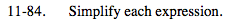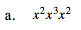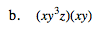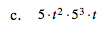### Home > MC2 > Chapter 11 > Lesson 11.3.1 > Problem11-84

11-84.To multiply expressionswith a common base, add
the exponents together.

$x^{(2+3+2)}$

$x^7$See (a).

$x^2y^4z$See (a) and (b).